# PSAT Math : How to find mode

## Example Questions

### Example Question #1 : How To Find Mode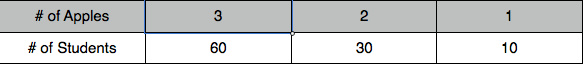Looking at the table, what is the difference of the mean minus the mode.

1.0

0.5

–1.0

–0.5

–0.5

Explanation:

The mode is 3 and subtracting 3 from 2.5 gives -0.5.

### Example Question #2 : How To Find Mode

Find the mode of the following set of numbers:

1, 6, 3, 6, 5, 9

6

5

8

9

6

Explanation:

Mode is the most frequently occuring number in a set. 6 appears most frequently since there are 2 sixes but the rest of the numbers only appear once. The mode is 6

### Example Question #3 : How To Find Mode

Find the mode in this set of numbers:

2, 100, 52, 97, 1, 7, 22, 19, 100

97

none of these

2

1

100

100

Explanation:

The mode is the number that appears the most

### Example Question #4 : How To Find Mode

Find the mode from the following set of numbers

1, 4, 8, 17, 8, 8, 15, 21, 32, 17

15

8

4

17

21

8

Explanation:

The mode is the number that appears the most in a given set of numbers

### Example Question #5 : How To Find Mode

Find the mode.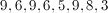Explanation:

The mode is the number that appears the most often. 9 appears three times.

### Example Question #6 : How To Find Mode

What is the mode of the following set of numbers?

24, 38, 26, 27, 7, 27, 34, 12, 13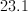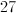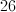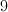Explanation:

The mode is the number that appears the most in a given set of numbers.

### Example Question #81 : Statistics

Consider the following dataset: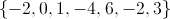The arithmetic mean of the dataset is.

The median of the dataset is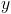.

The range of the dataset is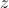.

The mode of the dataset is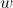.

Which of the following statements is true?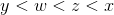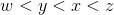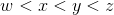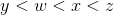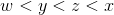Explanation:

In general: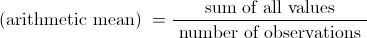In this case the mean is: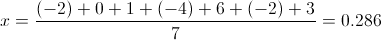To obtain the median, arrange the observations from smallest to largest: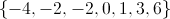When there is an odd number of observations, the median is the middle value. In this case: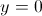The range is the difference between the largest and smallest value: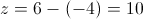The mode is the value that occurs most often in the dataset: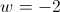Since: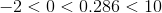we can conclude:### Example Question #82 : Statistics

 Marker Colors Students Blue 13 Pink 10 Orange 5 Brown 5 Green 7

The above chart shows the number of students in a class who chose each of the five marker colors available.

What is the mode of the above chart?Explanation:

The mode of a problem is the most common number, i.e. the number that appears the most times in a given set of data. In this case, only one number is repeated. Therefore, the mode is 5.

### Example Question #83 : Statistics

Find the mode(s) of the following set of numbers: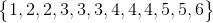andand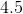andandExplanation:

The mode is the most frequently represented number in a data set. In this instance, that isand. There can be more than one mode.

### Example Question #84 : Statistics

Find the mode of the following set of numbers: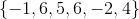The mode is the most frequently occurring number in a data set. In this instance, that is.